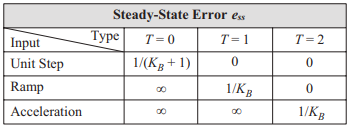where $$T$$ denotes the type of system, i.e., type 0, type 1, etc.
$$e_{ss}=\frac{1}{(K_B+1)}$$$$$e_{ss}=\frac{1}{(K_B+1)}$$$